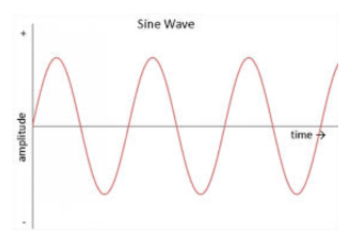# Problem: The amplitude of a soundwave can be expressed as a sin wave. Which of the following is an accurate description?A. A positive amplitude means the sound pressure level is higher B. A negative amplitude represents a rarefication of the baseline sound pressure in the air C. If the length of time expressed in the graph were 1 second, the frequency of the soundwave would be between 3 Hz and 3.5 Hz D. If the length of time expressed in the graph were 1 second, the frequency of the soundwave would be between 6 Hz and 7 Hz E. the sound is a pure tone

###### FREE Expert Solution

Periodic time:

We have 3.25 waves and total time of 1s.

T = 1s/3.25 = (1/3.25)s

f = 1/T = 1/(1/3.25)s = 3.25s-1(1Hz/1s-1) = 3.25Hz.

92% (254 ratings)###### Problem Details

The amplitude of a soundwave can be expressed as a sin wave. Which of the following is an accurate description?A. A positive amplitude means the sound pressure level is higher

B. A negative amplitude represents a rarefication of the baseline sound pressure in the air

C. If the length of time expressed in the graph were 1 second, the frequency of the soundwave would be between 3 Hz and 3.5 Hz

D. If the length of time expressed in the graph were 1 second, the frequency of the soundwave would be between 6 Hz and 7 Hz

E. the sound is a pure tone